# Ex: Determine if a Table Values Represents a Function

##By Mathispower4u

Ex: Determine if a Table Values Represents a Function# Recognizing functions from graphs

##By Khan Academy

Sal explains why a vertical line *doesn't* represent a function.# Recognizing functions from graphs

##By Khan Academy

Sal explains why a vertical line *doesn't* represent a function.# Representing functions as rules and graphs

##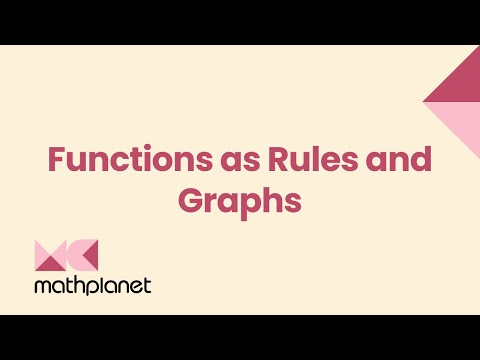By MathPlanetVideos

Write a rule for the function:# Recognizing functions from graphs

##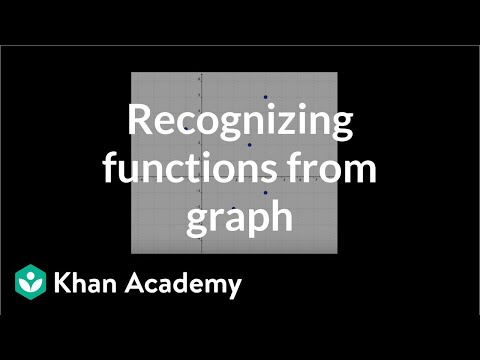By Khan Academy

Sal checks whether a given set of points can represent a function. For the set to represent a function, each domain element must have one corresponding range element at most.# Recognizing functions from graphs

##By Khan Academy

Sal checks whether a given set of points can represent a function. For the set to represent a function, each domain element must have one corresponding range element at most.# Comparing linear functions

##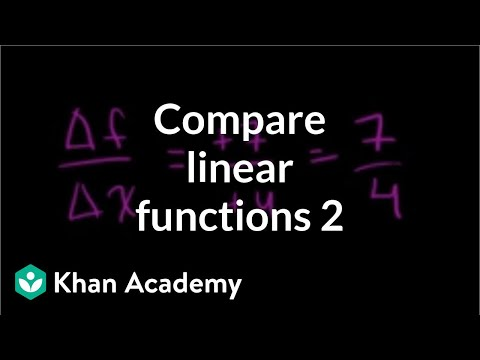By Khan Academy

Sal is given a table of values of a linear function and four linear graphs, and is asked to determine which graph has the same rate of change as the function represented in the table.# Comparing linear functions

##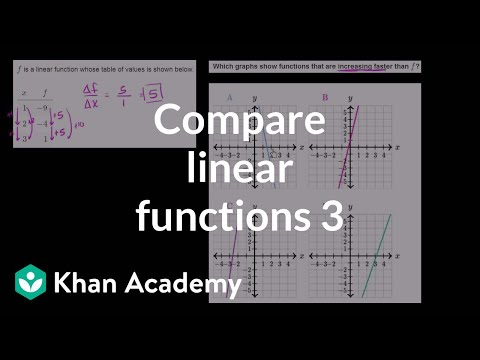By Khan Academy

Sal is given a table of values of a linear function and four linear graphs, and is asked to determine which graph increases faster than the function represented in the table.# Comparing linear functions

##By Khan Academy

Sal is given a table of values of a linear function and four linear graphs, and is asked to determine which graph increases faster than the function represented in the table.# Comparing linear functions

##By Khan Academy

Sal is given a table of values of a linear function and four linear graphs, and is asked to determine which graph has the same rate of change as the function represented in the table.# Constructing linear functions word problems

##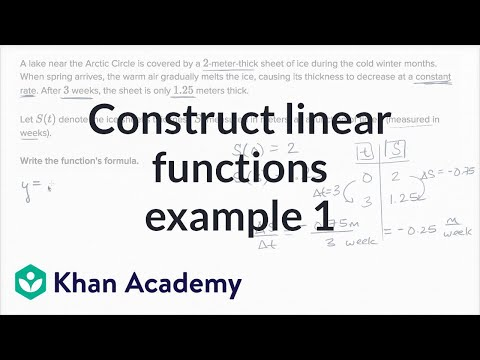By Khan Academy

Sal is given a verbal description of a real-world relationship involving a melting iceberg, and is asked to find the formula of the function that represents this relationship.# Recognizing functions from tables

##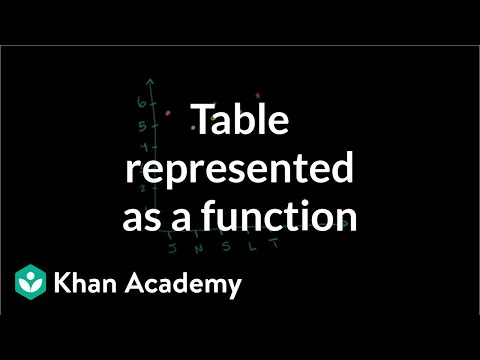By Khan Academy

Sal checks whether a table of people and their heights can represent a function that assigns a height to a name.# Find inverse values of functions

##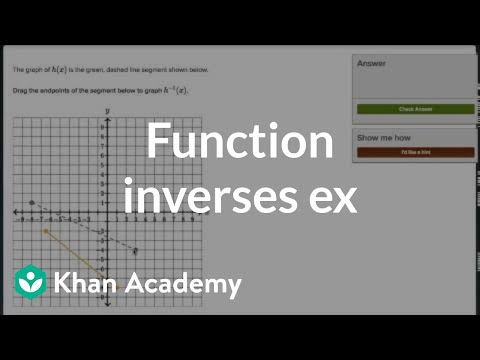By Khan Academy

Sal is given a line segment on the coordinate plane, and he graphs the inverse of the function represented by that segment.# Find inverse values of functions

##By Khan Academy

Sal is given a line segment on the coordinate plane, and he graphs the inverse of the function represented by that segment.# Find inverse values of functions

##By Khan Academy

Sal is given a line segment on the coordinate plane, and he graphs the inverse of the function represented by that segment.# Find inverse values of functions

##By Khan Academy

Sal is given a line segment on the coordinate plane, and he graphs the inverse of the function represented by that segment.# Comparing features of functions

##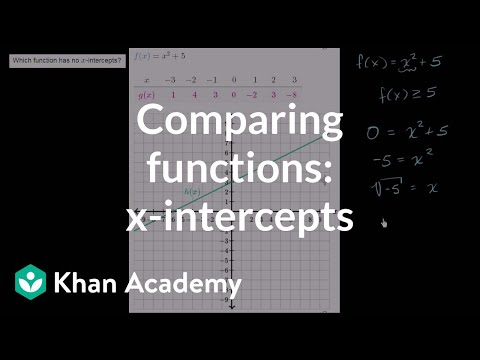By Khan Academy

Given several functions represented in different forms (as a formula, as a graph, and as a table of values), Sal finds the one with that has no x-intercepts.# Constructing linear functions word problems

##By Khan Academy

Sal is given a verbal description of a real-world relationship involving a melting iceberg, and is asked to find the formula of the function that represents this relationship.# Constructing linear functions word problems

##By Khan Academy

Sal is given a verbal description of a real-world relationship involving a melting iceberg, and is asked to find the formula of the function that represents this relationship.# Recognizing functions from tables

##By Khan Academy

Sal checks whether a table of people and their heights can represent a function that assigns a height to a name.